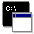## Firefly and PC GAMESS-related discussion club

Learn how to ask questions correctlyError in Multiplicity and number of electrons

Amir Nasser Shamkhali
amir_n_shamkhali@yahoo.com

Dear All
I have to optimize the structure of a Co containing complex with 3 or 1 unpaired electrons (Co2+). But I'm faced with a wonderful error:
TOTAL NUMBER OF SHELLS              =  202
TOTAL NUMBER OF BASIS FUNCTIONS     =  629
NUMBER OF ELECTRONS                 =  247
CHARGE OF MOLECULE                  =    0
STATE MULTIPLICITY                  =    1
NUMBER OF OCCUPIED ORBITALS (ALPHA) =  123
NUMBER OF OCCUPIED ORBITALS (BETA ) =  123
TOTAL NUMBER OF ATOMS               =   55
THE NUCLEAR REPULSION ENERGY IS     4124.6025430666

*** CHECK YOUR INPUT CHARGE AND MULTIPLICITY ***
THERE ARE   247 ELECTRONS, WITH CHARGE ICHARG=  0
BUT YOU SELECTED MULTIPLICITY MULT=  1
What can i do for this problem? I also attach my input file.This message contains the 4 kb attachment [ COB.inp ]Wed May 21 '14 6:11pmThis message read 914 times